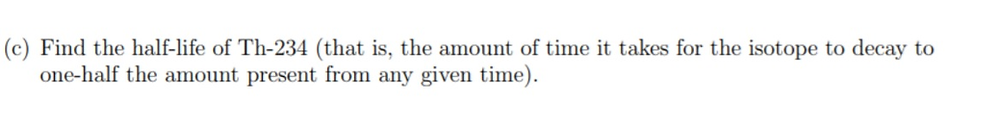# Separable Differential Equations: Exponential Decay

When I was in high school, my chemistry teacher presented me with a radioactive decay problem, and a formula that read:

$$Q=Q_{0} * e^{-r t}$$

Where (Q) represented the current amount of radioactive material, (Q_0) represented the starting amount of material, and (r) was some unknown “decay rate”. I was greatly confused by this. “Why do we raise the time function to the power of e?” I asked. “Why not raise the time function to the power of (frac{1} {2}) ? We are working with half-lives after all”. It turns out that the answer to this question is surprisingly nuanced, and requires a deep dive into differential equations. So let’s take a look at a radioactive decay problem through the lens of differential equations.Now I know this problem has four parts to it, but don’t panic—you won’t be needing your brown pants today. In fact, don’t even read Parts A-D yet. Let’s just look at the differential equation, and solve it before doing anything else:

$$frac{d Q}{d t}=-r Q$$

You get bonus points if you recognized this as a Separable Differential Equation, which means that you can move the two variables (Q and t) to opposite sides of the equation. The best way to succeed in a differential equations class is by always knowing what type of equation you’re working with. If you’re not sure what type of equation you’re looking at, you can always try to separate it. In this case, we multiply both sides by (dt), and divide both sides by (Q).

$$frac{d Q}{Q}=-r * d t$$

The point of separating the equation is so we can take the integral of both sides, thereby getting rid of the differential terms (dQ) and (dt).

$$int frac{d Q}{Q}=-r * int d t$$

Note that we can leave the (-r) term outside the integral for (t), since (r) is a constant, and does not depend on (t). We solve both integrals to get

$$ln (Q)=-r t+C_{1}$$

Never forget that (+C). Hopefully you kept those good habits from MAT21B. Also don’t forget why we’re doing this. “Solving” a differential equation means finding detailed temporal information about the time-sensitive variable, (Q(t)). When I write (Q(t)), I’m saying that “(Q) is a function of (t)”. Let’s finish up this equation:

$$Q(t)=e^{left(-r t+C_{1}right)}$$

Note that (e^{(-r t+C)}) can be written as: [(e^{-r t} * e^{C_{1}})]. Also note that (e^{C_{1}}) is just another constant, which I’ll call (C_2). That was a bit fast—let me do it again in slow motion:

$$Q(t)=e^{left(-r t+C_{1}right)}=e^{-r t} * e^{c_{1}}=C_{2} e^{-r t}$$

Great, now we’re almost done! The last thing that you have to deal with in any differential equation is getting rid of any lingering (C) The way that we do this is through Initial Conditions. Initial conditions allow us to plug in predetermined values for (Q) and (t) that reveal the value of (C_2). If you take a close look at the problem, you might notice that the wording for Part A gives us an initial condition right away. Let’s use it to find (C_2):From this phrasing, and our equation: (Q(t)=C_{2} e^{-r t}), we get:

$$Q(0)=C_{2} e^{-r(0)}=C_{2}=100 mathrm{mg}$$

And there you have it. Now that we know (C_2), we can write our solved differential equation.

$$boldsymbol{Q}(boldsymbol{t})=mathbf{1} mathbf{0} mathbf{0} boldsymbol{m} boldsymbol{g} * boldsymbol{e}^{-boldsymbol{r} t}$$

I know I haven’t solved any of Parts A-D yet, but now that you have the finished equation, they will basically solve themselves! Here we go:

### Part AHere we are asked to find (r) if (Q(1 week) = 84 mathrm(mg)). Let’s plug it in:

$$begin{array}{c}{Q(1)=84 m g=100 m g * e^{-r}} \ {e^{-r}=0.84} \ {r=-ln (0.84)=0.1744}end{array}$$

To make sure that units are consistent, the units of (r) must be (frac {1} {weeks}). Otherwise, (e^{-rt}) would have non-cancelling units, and that would be awkward.

### Part BWe practically already solved this one; just plug in (r) from Part A:

$$Q(t)=100 m g * e^{-0.1744 t}$$

### Part CThis time, we’re looking for (t) when (Q(t) = 50mg ). Again, just plug it in:

begin{array}{c}{Q(t)=50 m g=100 m g * e^{-0.1744 t}} \ {e^{-0.1744 t}=0.5} \ {t=-frac{ln (0.5)}{0.1744}=3.974}end{array}

Quick, what units does (t) have? Hopefully you said “weeks”. We have to remain consistent with our given values.

### Part DThe only tricky thing going on here is labelling the two points. We know 3 points easily: (Q(0)=100 m g, Q(1)=84 m g), and (Q(3.974)=50 m g). If you want to be fancy you include: (Qleft(frac{1}{0.1774}right)=frac{100}{e} m g), or (Q(2 * 3.974)=25 m g). The following graph is a reasonable solution:### What Did We Learn?

Aside from how to solve the above problem, we learned two important things today: first of all, that solving a differential equation should always be your first step in tackling a problem, regardless of how many parts the question has. Additionally, we learned that the equation [ (Q=Q_{0} * e^{-r t}) ] is the ultimate solution to exponential decay problems—problems in which the rate of change of a substance, (frac{d Q}{d t}), is proportional to how much of that substance you have. When I was given this equation in high school, I didn’t understand why we used the natural number (e). But knowing that the equation represents the general case of this differential equation completely explains why mathematicians prefer it over all other potential solutions for such a problem.## Jonathan Brand

December 10, 2019

View Profile

#### Get in Touch

Thanks! We'll reach out shortly.